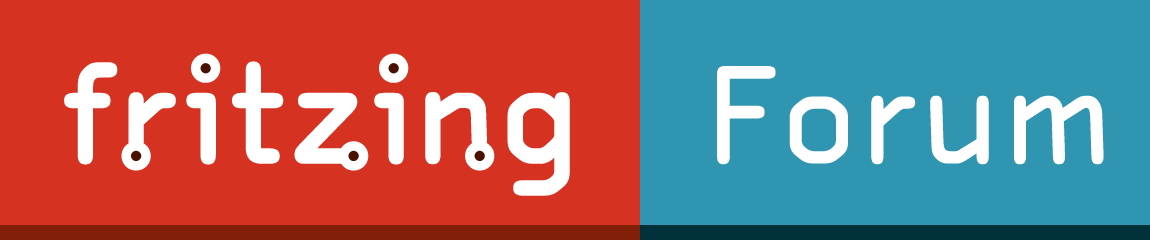# Constraint design of PCB board

Hi,

I have a PCB board with a specific design. Specific shape, cuts, and connector location.

(I designed it as an SVG file.)

I tried to use the image as board image but I can’t see the connector.
If I use it as a component, I don’t have the shape/cuts…

how would you do ?

Mayby this vid will tell you how to fix custom PCBs

That sounds like you are mixing up (together) the functions of a part (component) and pcb. An svg file used for a pcb image contains only cutout shapes and (maybe silkscreen). It will not have anything related to connectors or copper.

A part (component) has the copper pads, silkscreen, and (optionally) drill holes. The holes can be for through hole connections (when they have a copper border (svg stroke width), or mounting holes when not stroke exists. A common recommendation is to mark mounting hole location of a part using only silkscreen. That way the part can be used without the mounting hole when desired, and the silkscreen shows where to place a separate “hole” component when it is wanted.

You need a board svg, and a separate part (or multiple parts).

Ok, thanks. That’s a bit what I reached.
The only tricky thing is to align board and part.

Only tricky because the board does not do align to grid. Suggest putting a marker (silkscreen) on the board someplace that will be on the grid. Place a part (like a hole), align it to grid, then drag the board to match. Lock the board to keep it from moving, delete the part used to align the board, then place the real part.

To align connector on the grid, position the connectors on exact measures, like.

That works for aligning a connector (that is in a part) to the grid of the current view, or connectors within a part relative to each other. It does not work for aligning board image with the grid. If the image is not aligned to the grid, the connector of the part will not be aligned with the image either.

You can place the board image using exact coordinates, but you need to know what (if any) the offset is from the image reference point (corner) to the connector position that is to be lined up with the grid. That offset will be consistent for a single board image, but could easily be different for 2 different boards. once the board image is positioned correctly (and locked) then parts can be positioned using the standard snap (align to grid), without needing to be placed by exact coordinates.

(post withdrawn by author, will be automatically deleted in 24 hours unless flagged)

Right, that’s a complex topic. Let’s do an example with a good mix of metrics and imperial systems.

• Card dimensions: 70mm x 60mm
• 2 Mounting holes of 4mm at 10mm of the borders in each corners.
• 10 connectors, 1mil spaced on each longest side at 2inches of each other, hole diameter 1mil copper size 0.5mil, first hole at 23mm of a border

Can you complete the svg file, add others to create a component … ?
thanks

For 72dpi, the SVG would be but it’s not aligned in Fritzing:

``````<?xml version='1.0' encoding='UTF-8' standalone='no'?>
<svg baseProfile='tiny'
height="60mm"
version='1.2'
viewBox='0 0 1984.25 1700.78'
width='70mm'
x="0"
xmlns='http://www.w3.org/2000/svg'
y="0">
<g id="silkscreen">
<rect x="0" y="0" width="1984.25" height="1700.78" fill="none" stroke="#000000" stroke-width="1"/>
<circle cx="283.46" cy="283.46" fill="black" id="nonconn0" r="56.69" stroke="#f9a435" stroke-width="0"/>
<circle cx="1700.79" cy="283.46" fill="black" id="nonconn0" r="56.69" stroke="#f9a435" stroke-width="0"/>
<circle cx="283.46" cy="1417.32" fill="black" id="nonconn0" r="56.69" stroke="#f9a435" stroke-width="0"/>
<circle cx="1700.79" cy="1417.32" fill="black" id="nonconn0" r="56.69" stroke="#f9a435" stroke-width="0"/>
</g>
<g id="copper0">
<circle cx="651.96" cy="130.39" fill="none" id="pin1.1" r="15.59" stroke="#f9a435" stroke-width="7.08"/>
<circle cx="723.96" cy="130.39" fill="none" id="pin1.1" r="15.59" stroke="#f9a435" stroke-width="7.08"/>
<circle cx="795.96" cy="130.39" fill="none" id="pin1.1" r="15.59" stroke="#f9a435" stroke-width="7.08"/>
<circle cx="867.96" cy="130.39" fill="none" id="pin1.1" r="15.59" stroke="#f9a435" stroke-width="7.08"/>
<circle cx="939.96" cy="130.39" fill="none" id="pin1.1" r="15.59" stroke="#f9a435" stroke-width="7.08"/>
<circle cx="1011.96" cy="130.39" fill="none" id="pin1.1" r="15.59" stroke="#f9a435" stroke-width="7.08"/>
<circle cx="1083.96" cy="130.39" fill="none" id="pin1.1" r="15.59" stroke="#f9a435" stroke-width="7.08"/>
<circle cx="1155.96" cy="130.39" fill="none" id="pin1.1" r="15.59" stroke="#f9a435" stroke-width="7.08"/>
<circle cx="1227.96" cy="130.39" fill="none" id="pin1.1" r="15.59" stroke="#f9a435" stroke-width="7.08"/>
<circle cx="1299.96" cy="130.39" fill="none" id="pin1.1" r="15.59" stroke="#f9a435" stroke-width="7.08"/>
<circle cx="651.96" cy="1570.39" fill="none" id="pin1.1" r="15.59" stroke="#f9a435" stroke-width="7.08"/>
<circle cx="723.96" cy="1570.39" fill="none" id="pin1.1" r="15.59" stroke="#f9a435" stroke-width="7.08"/>
<circle cx="795.96" cy="1570.39" fill="none" id="pin1.1" r="15.59" stroke="#f9a435" stroke-width="7.08"/>
<circle cx="867.96" cy="1570.39" fill="none" id="pin1.1" r="15.59" stroke="#f9a435" stroke-width="7.08"/>
<circle cx="939.96" cy="1570.39" fill="none" id="pin1.1" r="15.59" stroke="#f9a435" stroke-width="7.08"/>
<circle cx="1011.96" cy="1570.39" fill="none" id="pin1.1" r="15.59" stroke="#f9a435" stroke-width="7.08"/>
<circle cx="1083.96" cy="1570.39" fill="none" id="pin1.1" r="15.59" stroke="#f9a435" stroke-width="7.08"/>
<circle cx="1155.96" cy="1570.39" fill="none" id="pin1.1" r="15.59" stroke="#f9a435" stroke-width="7.08"/>
<circle cx="1227.96" cy="1570.39" fill="none" id="pin1.1" r="15.59" stroke="#f9a435" stroke-width="7.08"/>
<circle cx="1299.96" cy="1570.39" fill="none" id="pin1.1" r="15.59" stroke="#f9a435" stroke-width="7.08"/>
<g id="copper1">
<circle cx="651.96" cy="130.39" fill="none" id="pin1.1" r="15.59" stroke="#f9a435" stroke-width="7.08"/>
<circle cx="723.96" cy="130.39" fill="none" id="pin1.1" r="15.59" stroke="#f9a435" stroke-width="7.08"/>
<circle cx="795.96" cy="130.39" fill="none" id="pin1.1" r="15.59" stroke="#f9a435" stroke-width="7.08"/>
<circle cx="867.96" cy="130.39" fill="none" id="pin1.1" r="15.59" stroke="#f9a435" stroke-width="7.08"/>
<circle cx="939.96" cy="130.39" fill="none" id="pin1.1" r="15.59" stroke="#f9a435" stroke-width="7.08"/>
<circle cx="1011.96" cy="130.39" fill="none" id="pin1.1" r="15.59" stroke="#f9a435" stroke-width="7.08"/>
<circle cx="1083.96" cy="130.39" fill="none" id="pin1.1" r="15.59" stroke="#f9a435" stroke-width="7.08"/>
<circle cx="1155.96" cy="130.39" fill="none" id="pin1.1" r="15.59" stroke="#f9a435" stroke-width="7.08"/>
<circle cx="1227.96" cy="130.39" fill="none" id="pin1.1" r="15.59" stroke="#f9a435" stroke-width="7.08"/>
<circle cx="1299.96" cy="130.39" fill="none" id="pin1.1" r="15.59" stroke="#f9a435" stroke-width="7.08"/>
<circle cx="651.96" cy="1570.39" fill="none" id="pin1.1" r="15.59" stroke="#f9a435" stroke-width="7.08"/>
<circle cx="723.96" cy="1570.39" fill="none" id="pin1.1" r="15.59" stroke="#f9a435" stroke-width="7.08"/>
<circle cx="795.96" cy="1570.39" fill="none" id="pin1.1" r="15.59" stroke="#f9a435" stroke-width="7.08"/>
<circle cx="867.96" cy="1570.39" fill="none" id="pin1.1" r="15.59" stroke="#f9a435" stroke-width="7.08"/>
<circle cx="939.96" cy="1570.39" fill="none" id="pin1.1" r="15.59" stroke="#f9a435" stroke-width="7.08"/>
<circle cx="1011.96" cy="1570.39" fill="none" id="pin1.1" r="15.59" stroke="#f9a435" stroke-width="7.08"/>
<circle cx="1083.96" cy="1570.39" fill="none" id="pin1.1" r="15.59" stroke="#f9a435" stroke-width="7.08"/>
<circle cx="1155.96" cy="1570.39" fill="none" id="pin1.1" r="15.59" stroke="#f9a435" stroke-width="7.08"/>
<circle cx="1227.96" cy="1570.39" fill="none" id="pin1.1" r="15.59" stroke="#f9a435" stroke-width="7.08"/>
<circle cx="1299.96" cy="1570.39" fill="none" id="pin1.1" r="15.59" stroke="#f9a435" stroke-width="7.08"/>
</g>
</g>
</svg>``````